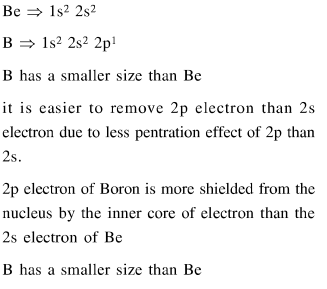# B has a smaller first ionization enthalpy than Be. Consider the following statements :

Question:

B has a smaller first ionization enthalpy than Be. Consider the following statements :

(I) It is easier to remove $2 \mathrm{p}$ electron than $2 \mathrm{~s}$ electron

(II) $2 \mathrm{p}$ electron of $\mathrm{B}$ is more shielded from the nucleus by the inner core of electrons than the $2 \mathrm{~s}$ electrons of $\mathrm{Be}$.

(III) 2 s electron has more penetration power than 2 p electron.

(IV) atomic radius of $\mathrm{B}$ is more than $\mathrm{Be}$

(Atomic number $\mathrm{B}=5, \mathrm{Be}=4$ )

The correct statements are :

1. (I), (II) and (III)

2. (II), (III) and (IV)

3. (I), (III) and (IV)

4. (I), (II) and (IV)

Correct Option: 1

Solution: